# Solving Quadratic Equations 9 7 by Using Square

• Slides: 11Solving Quadratic Equations 9 -7 by Using Square Roots Objective Solve quadratic equations by using square roots. Holt Mc. Dougal Algebra 1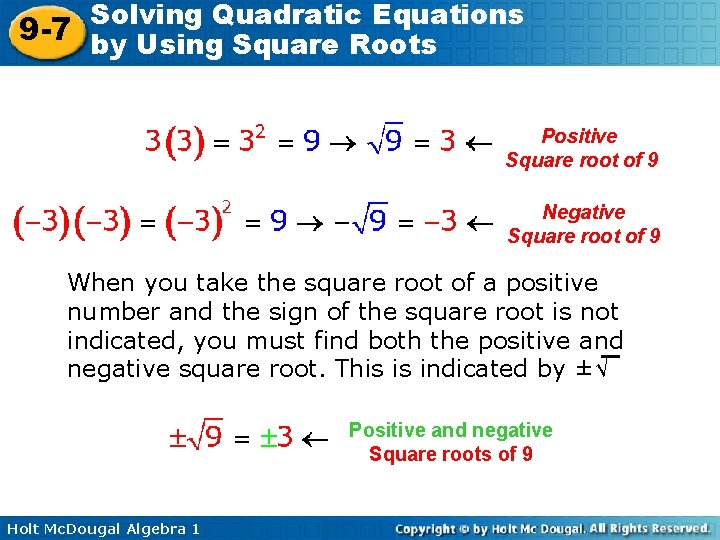Solving Quadratic Equations 9 -7 by Using Square Roots Positive Square root of 9 Negative Square root of 9 When you take the square root of a positive number and the sign of the square root is not indicated, you must find both the positive and negative square root. This is indicated by ±√ Positive and negative Square roots of 9 Holt Mc. Dougal Algebra 1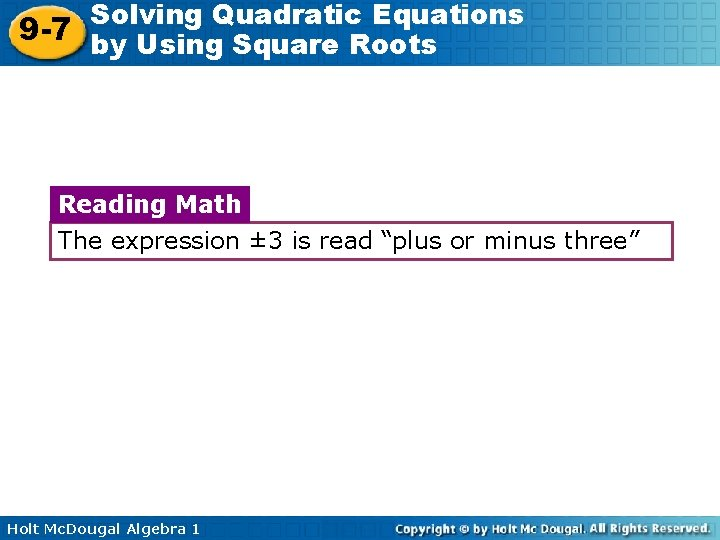Solving Quadratic Equations 9 -7 by Using Square Roots Reading Math The expression ± 3 is read “plus or minus three” Holt Mc. Dougal Algebra 1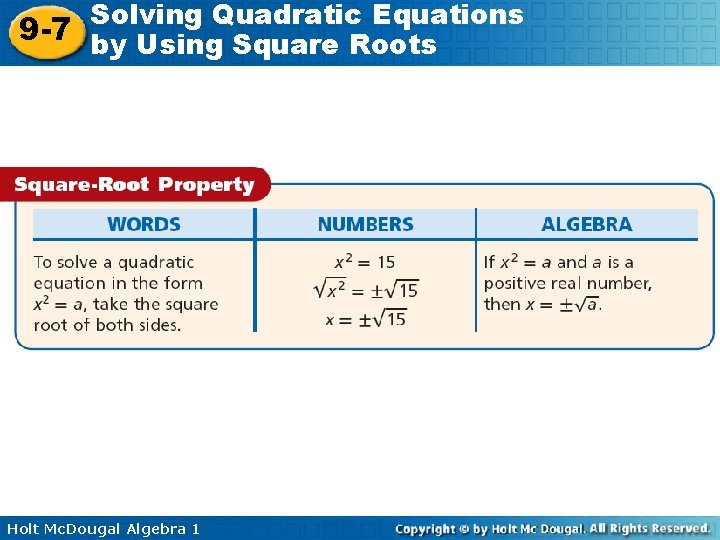Solving Quadratic Equations 9 -7 by Using Square Roots Holt Mc. Dougal Algebra 1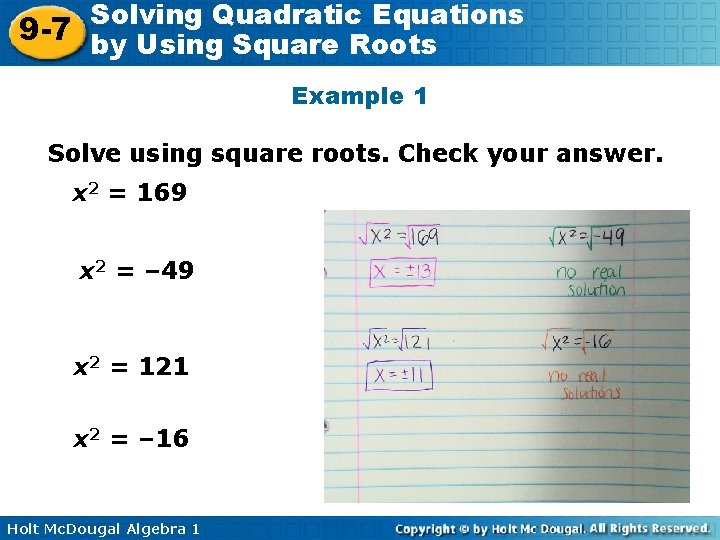Solving Quadratic Equations 9 -7 by Using Square Roots Example 1 Solve using square roots. Check your answer. x 2 = 169 x 2 = – 49 x 2 = 121 x 2 = – 16 Holt Mc. Dougal Algebra 1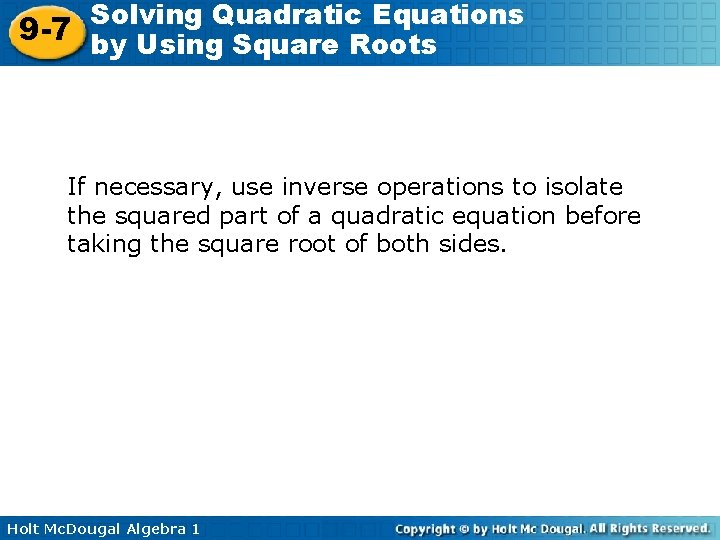Solving Quadratic Equations 9 -7 by Using Square Roots If necessary, use inverse operations to isolate the squared part of a quadratic equation before taking the square root of both sides. Holt Mc. Dougal Algebra 1Solving Quadratic Equations 9 -7 by Using Square Roots Example 2 Solve using square roots. x 2 + 7 = 7 16 x 2 – 49 = 0 Holt Mc. Dougal Algebra 1Solving Quadratic Equations 9 -7 by Using Square Roots Example 3 Solve by using square roots. Check your answer. 100 x 2 + 49 = 0 (x – 5)2 = 16 Holt Mc. Dougal Algebra 1Solving Quadratic Equations 9 -7 by Using Square Roots When solving quadratic equations by using square roots, you may need to find the square root of a number that is not a perfect square. In this case, the answer is an irrational number. You can approximate the solutions. Holt Mc. Dougal Algebra 1Solving Quadratic Equations 9 -7 by Using Square Roots Example 4 Solve. Round to the nearest hundredth. x 2 = 15 – 3 x 2 + 90 = 0 0 = 90 – x 2 + 45 = 0 Holt Mc. Dougal Algebra 1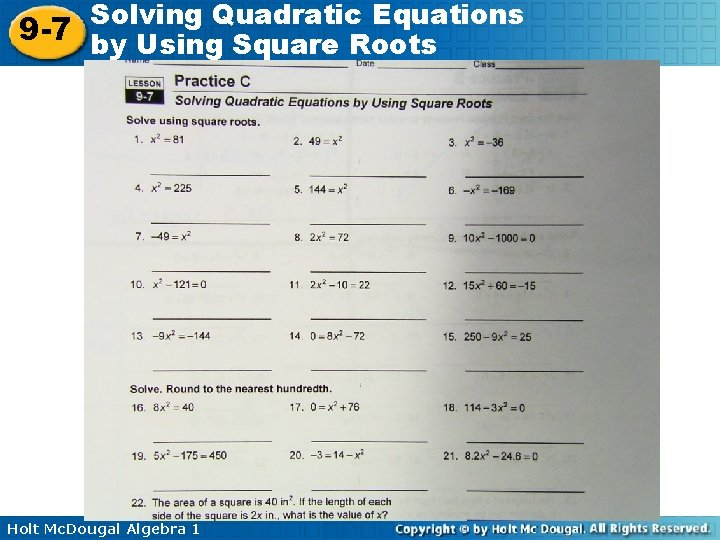Solving Quadratic Equations 9 -7 by Using Square Roots Holt Mc. Dougal Algebra 1